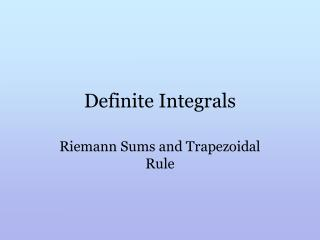# Definite Integrals - PowerPoint PPT PresentationDownload PresentationDefinite Integrals

Definite Integrals
Download Presentation## Definite Integrals

- - - - - - - - - - - - - - - - - - - - - - - - - - - E N D - - - - - - - - - - - - - - - - - - - - - - - - - - -
##### Presentation Transcript

1. Definite Integrals Riemann Sums and Trapezoidal Rule

2. Why all this work? Why learn integration? We are now going to look to find the numerical value of any curve f(x) from a to b, bounded by the x axis. (Fig 1) The connection to integration that will be explained tomorrow. Right now we will concentrate on some of the methods that can be used to find the area under any curve.

3. Method 1: Riemann Sums (See Fig 2) If we partition a particular function into intervals from x0 (a) to xn (b) with evenly spaced subintervals (xk, xk+1, xk+2…) we could find the area under the curve IF we could find the area of all those little subintervals. The more subintervals, the more accurate the area, right? Lets look at these as rectangles.

4. Riemann Sums (cont.) (Fig. 3) If we choose a particular number, c, in each subinterval, f(c)·Δx = the area of the particular rectangle. If we add all these rectangles, we’ll get a pretty close approximation of the total area under the curve. Notice: Arectangle = length·width = f(c)·Δx

5. Riemann Sums (cont.) Whether we use the left side of the rectangle or the right side we can see that the more rectangles the more accurate the area. Here’s another adds all the rectangles. Area under curve =

6. Trapezoidal Rule (Fig.4 ) Instead of rectangles, we look at the subintervals as trapezoids. Again, the more trapezoids you put in, the more accurate the estimation. What is the formula for the area of a trapezoid?

7. For just one trapezoid If I add all the trapezoids (n of them) n=number of sub intervals

8. Will we do this all the time now? Good grief NO!! This is a very rote method. But as you see from the illustrations, the more intervals you have the more accurately you can find the area

9. Example • Use trapezoidal rule to partition into 4 subintervals to estimate TONIGHT’S PROBLEM Use trapezoidal rule to partition into 4 subintervals to estimate